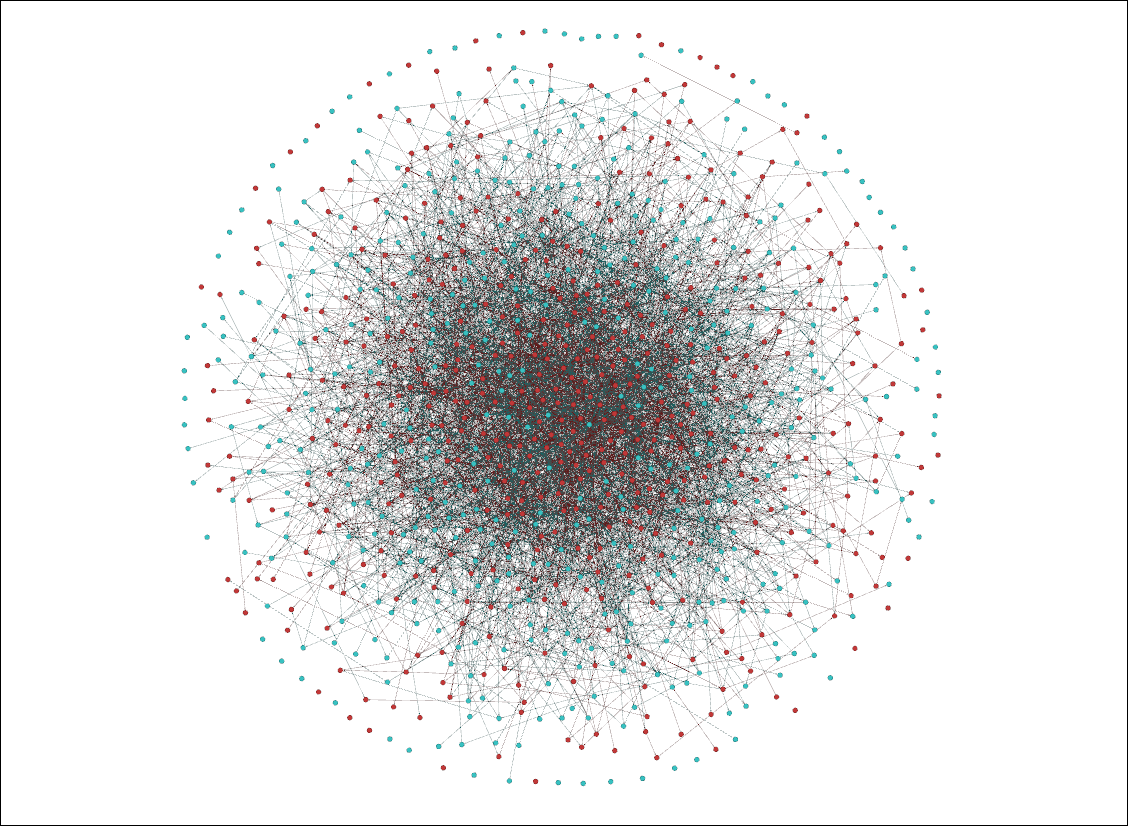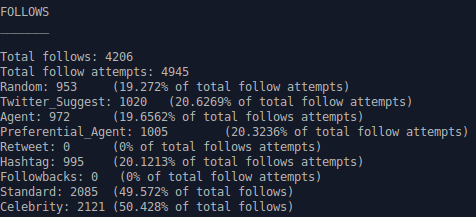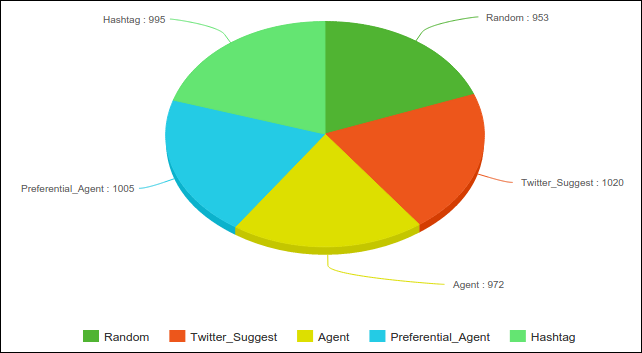hashkat.org

The twitter follow model is a combination of the other follow_models with different weights that can be set by the user. This potentially results in a network where some follows are random, some are based on which agent has the highest number of followers, some are based on what the agents's type is, some are based on the number of followers an agent of a particular agent type has, and some are based on the hashtags present in tweets. Undeniably the most complex of all the follow models, we will create a twitter follow_model simulation based on what we've learned in the previous tutorials. The input file that we will create in this tutorial can be found for reference in /hashkat/docs/tutorial_input_files/tutorial08/. This tutorial should take approximately 10 minutes to complete.

#### Constructing The Network

As always we start with the INFILE.yaml created in the previous tutorial.

Set:

Since the twitter follow model is a combination of the other follow models, we have to give the models weights. We would like to have all the follow models weighted equally for this simulation, therefore, every follow model will have a weight of 1.0 to accomplish this. #k@ will sum the weights and divide to give the probability, 1/5 or 20%. We could have accomplished the same result by giving each model a weight of 0.2.

``python follow_model: # See notes above twitter

model_weights: {random: 1.0, twitter_suggest: 1.0, agent: 1.0, preferential_agent: 1.0, hashtag: 1.0} ```

It is possible to exclude one or more models by giving it a weight of 0.0.

#### Running and Visualizing The Network

We produced the following visualization in Gephi:The combination of all the follow models produces an interesting network. Due to the presence of the 'agent' and 'preferential_agent' follow models, we can see that 'Celebrity' agents (red nodes) are the more connected agents, though there are highly connected 'Standard' agents as well. The random follow model may lead to a lucky 'Standard' agent gaining followers and the 'twitter_suggest' (or preferential_global) follow_model with preferential attachment compounding their gain.

Due to the presence of all the other follow models, it is difficult to notice the presence of the hashtag follow model. The hashtag preference doesn't affect 100% of the agent's follows, so the effect seems muted, unlike the polarization seen in the previous tutorial.

Looking into our main_stats.dat output file, we can see that every follow_model was used in this network simulation as shown below:The graph below compares the weights of the follow models in the network after the simulation has been run.We can see that the 4855 total weight corresponds to post simulation weights of: random 0.196, twitter_suggest (preferential_global) 0.21, agent 0.20, preferential_agent 0.207, and hashtag 0.205.

Note: this pie graph was constructed using meta-chart.com/pie.

#### Next Steps

With the completion of this and previous tutorials, you have familiarized yourself with each of the follow models offered by #k@. You now have the fundamental knowledge necessary to create a variety of different network simulations using this program. We shall now proceed to some of the more advanced aspects of #k@ to enable you to produce the most realistic simulations possible.